## Pentaflake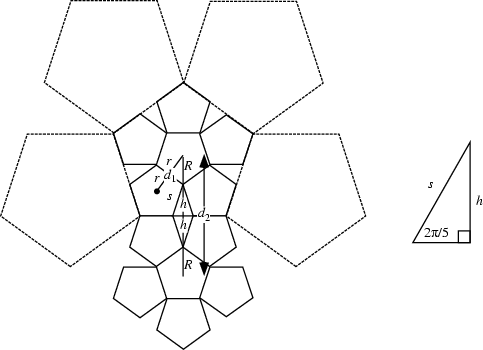A Fractal with 5-fold symmetry. As illustrated above, five Pentagons can be arranged around an identical Pentagon to form the first iteration of the pentaflake. This cluster of six pentagons has the shape of a pentagon with five triangular wedges removed. This construction was first noticed by Albrecht Dürer (Dixon 1991).

For a pentagon of side length 1, the first ring of pentagons has centers at Radius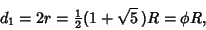(1)

whereis the Golden Ratio. The Inradiusand Circumradiusare related by(2)

and these are related to the side lengthby(3)

The height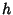is(4)

giving a Radius of the second ring as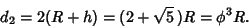(5)

Continuing, theth pentagon ring is located at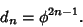(6)

Now, the length of the side of the first pentagon compound is given by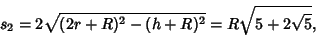(7)

so the ratio of side lengths of the original pentagon to that of the compound is(8)

We can now calculate the dimension of the pentaflake fractal. Letbe the number of black pentagons andthe length of side of a pentagon after theiteration,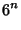(9)(10)

The Capacity Dimension is therefore(11)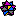Weisstein, E. W. Fractals.'' Mathematica notebook Fractal.m.